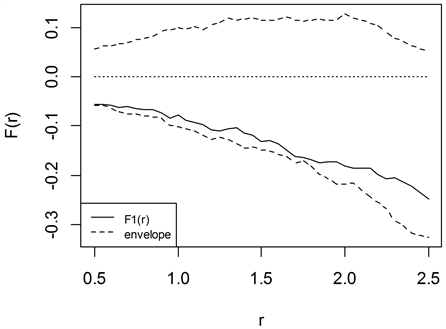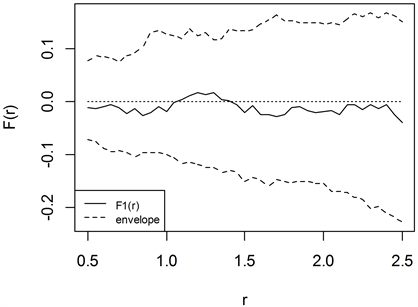﻿ 高速公路交通流量的齐次泊松过程模型检验 Test for the Homogeneous Poisson Process Model of Highway Traffic Flows

Statistics and Application
Vol.06 No.05(2017), Article ID:23244,7 pages
10.12677/SA.2017.65065

Test for the Homogeneous Poisson Process Model of Highway Traffic Flows

Ya’nan Li, Shuhe Lei*, Fenglin Sun

School of Mathematical Sciences, Ocean University of China, Qingdao Shandong

*通讯作者。

Email: *lyn_stat@163.com

Received: Dec. 7th, 2017; accepted: Dec. 22nd, 2017; published: Dec. 29th, 2017ABSTRACT

In order to build a traffic model of vehicles arrival times of highway, using the observed traffic data, a stochastic point pattern is composed by recording vehicles arrival times. Based on the theory of spatial point process, the Ripley’s K function is applied to one-dimensional stochastic point process. According to the characteristics of Homogeneous Poisson Process, a graphical approach is proposed to test Homogeneous Poisson Process combined with envelope test. And the effectiveness of the test is verified by simulation. Then the graphical approach is applied to the patterns, and the result indicates that arrival times of vehicles composed a Homogeneous Poisson Process. Finally, the traffic model of Chang-Tai freeway is obtained by estimating the parameter of the Homogeneous Poisson Process.

Keywords:Homogeneous Poisson Process, Traffic Flows, K Function, Envelope Test

Email: *lyn_stat@163.com1. 引言

2. 理论与方法

2.1. 空间点过程与Ripley的K函数

$K\left(r\right)=\frac{1}{\lambda }E\left[{N}_{r}\left(x\right)\right],\text{\hspace{0.17em}}r>0$ (1)

$K\left(r\right)=\pi \cdot {r}^{2},\text{\hspace{0.17em}}r>0$ (2)

 (3)

$\stackrel{^}{K}\left(r\right)=\frac{1}{\stackrel{^}{\lambda }}\frac{\underset{i=1}{\overset{N\left(\phi \right)}{\sum }}\underset{j=1}{\overset{N\left(\phi \right)}{\sum }}I\left\{|{x}_{i}-{x}_{j}| (4)

$K\left(r\right)=2r$ (5)

2.2. 图形化检验方法

$\mathrm{log}\left\{{\beta }^{n}\mathrm{exp}\left[-\beta \underset{i=1}{\overset{n}{\sum }}{T}_{i}\right]\mathrm{exp}\left[-\beta \left(T-{t}_{n}\right)\right]\right\}=n\mathrm{log}\beta -\beta T$ (6)

$\stackrel{^}{\beta }=n/T$ (7)

${F}_{i}\left(r\right)={\stackrel{^}{K}}_{i}\left(r\right)-{K}_{0}\left(r\right)$ (8)

${F}_{0}\left(r\right)=0$ ，并定义上下包络为：

$U\left(r\right)=\mathrm{max}\left\{{F}_{i}\left(r\right)\right\},\text{\hspace{0.17em}}\text{\hspace{0.17em}}i=2,3,\cdots ,s+1$ (9)

$L\left(r\right)=\mathrm{min}\left\{{F}_{i}\left(r\right)\right\},\text{\hspace{0.17em}}\text{\hspace{0.17em}}i=2,3,\cdots ,s+1$ (10)

$L\left(r\right)<{F}_{i}\left(r\right) , ${r}_{\mathrm{min}} (11)

2.3. 随机模拟

3. 实证分析

$t=m-\frac{p}{v}$ (12)Figure 1. The envelope test of Homogeneous Poisson ProcessFigure 2. The envelope test of the arrival times in Chang-tai Highway

4. 结论与讨论

Test for the Homogeneous Poisson Process Model of Highway Traffic Flows[J]. 统计学与应用, 2017, 06(05): 576-582. http://dx.doi.org/10.12677/SA.2017.65065

1. 1. 郭冠英. 交通工程普及讲座第九讲交通量的到达分布[J]. 华东公路, 1983(5): 63-73.

2. 2. 莫智锋, 余嘉, 孙跃. 基于泊松分布的微观交通仿真断面发车数学模型研究[J]. 城市交通, 2002(4): 4-6.

3. 3. 丁春蕾. 高速公路交通流量数学模型与分析[D]: [硕士学位论文]. 天津: 天津大学, 2003: 4-7.

4. 4. 张蓉, 石建军, 任福田, 等. 数理统计知识在交通仿真中的部分应用[J]. 北京工业大学学报, 2003, 29(4): 473- 476.

5. 5. 邓永录, 梁之舜. 随机点过程及其应用[M]. 北京: 科学出版社, 1992: 106-109.

6. 6. 薛珊荣. “泊松分布”在交通工程中的应用[J]. 公路, 1981(1): 26-30.

7. 7. Dixon, P.M. (2006) Ripley’s K Function. Encyclopedia of Environmetrics, 3, 1796-1803.

8. 8. 郭明旻. 交通实测视频与数据共享平台[EB/OL]. http://traflow.fudan.edu.cn/instr.html, 2017-04-05.

9. 9. Baddeley, A. (2007) Spatial Point Processes and Their Applications. Stochastic Geometry, 1, 1-75.

10. 10. Stoyan, D. and Penttinen, A. (2000) Recent Applications of Point Process Methods in Forestry Statistics. Statistical Science, 15, 61-78.

11. 11. Grabarnik, P., Myllymäki, M. and Stoyan, D. (2011) Correct Testing of Mark Independence for Marked Point Patterns. Ecological Modelling, 222, 3888-3894. https://doi.org/10.1016/j.ecolmodel.2011.10.005

12. 12. 许谦, 郭明旻, 吴正. 嘉兴两条城际高速公路车速测量与统计研究[J]. 水动力学研究与进展, 2014, 29(3): 325-331.